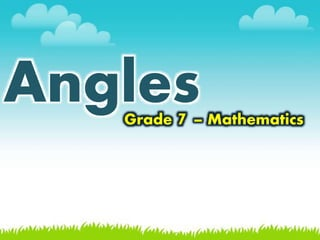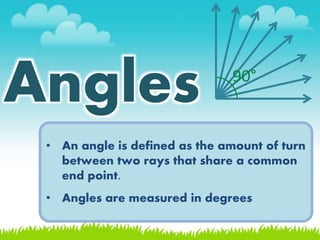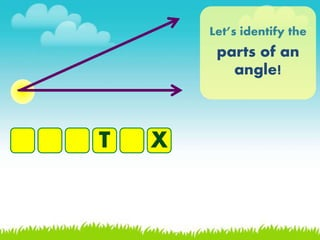Se está descargando tu SlideShare. ×Cargando en…3
×

1 de 40
1 de 40

# Angles

This preview may not appear the same on the actual version of the PPT slides.
Some formats may change due to font and size settings available on the audience's device.

Inclusions of the file attachment:
* Fonts used
* Soft copy of the WHOLE ppt slides with effects

ACCEPTING COMMISSIONED POWERPOINT SLIDES
ACCEPTING COMMISSIONED POWERPOINT SLIDES
ACCEPTING COMMISSIONED POWERPOINT SLIDES
EMAIL queenyedda@gmail.com
- - - - - - - - - - - - -
- Definition of Angles
- Parts of Angles
- Protractor
- Kinds of Angles
- Measuring Angles

The Assignment on the last slide is for them to have a background on the next lesson.

This preview may not appear the same on the actual version of the PPT slides.
Some formats may change due to font and size settings available on the audience's device.

Inclusions of the file attachment:
* Fonts used
* Soft copy of the WHOLE ppt slides with effects

ACCEPTING COMMISSIONED POWERPOINT SLIDES
ACCEPTING COMMISSIONED POWERPOINT SLIDES
ACCEPTING COMMISSIONED POWERPOINT SLIDES
EMAIL queenyedda@gmail.com
- - - - - - - - - - - - -
- Definition of Angles
- Parts of Angles
- Protractor
- Kinds of Angles
- Measuring Angles

The Assignment on the last slide is for them to have a background on the next lesson.

### Angles

1. 1. AnglesGrade 7 – Mathematics
2. 2. • An angle is defined as the amount of turn between two rays that share a common end point. • Angles are measured in degrees Angles 90°
3. 3. Let’s identify the parts of an angle! T X
4. 4. Let’s identify the parts of an angle! V E R T E X
5. 5. Let’s identify the parts of an angle! R
6. 6. Let’s identify the parts of an angle! A R M S
7. 7. Let’s identify the parts of an angle! D G
8. 8. Let’s identify the parts of an angle! D E G R E E S
9. 9. the common point where the two rays meet vertex the rays that make up the angle arms the measure of the size of an angle degrees
10. 10. Naming Angles 1 One way is to use the ∠ symbol followed by three letters. The first and third letters indicate points on the two arms. The letter in the middle is the vertex. ∠ __ __ __ A B C ∠ __ __ __ C B A
11. 11. Naming Angles using the ∠ symbol only with the vertex point. The angle would be called ∠B. by using the ∠ symbol followed by the letter or number between the angle. This would be ∠1. 1 ∠ABC ∠CBA ∠CAB ∠BCA ∠B ∠1
12. 12. 4 5 ? ? ? ? ? ? ? ? ?
13. 13. Kinds of Angles acute angle less than 90° obtuse angle more than 90° less than 180° right angle exactly to 90°
14. 14. Kinds of Angles reflex angle more than 180° Full rotation exactly 360° straight angle exactly to 180°
15. 15. acute right obtuse straight
16. 16. Protractor A tool that is used to measure angles. outer scale inner scale center
17. 17. Measuring Angles Place the center of the protractor on the vertex B A B C
18. 18. Measuring Angles Place the center of the protractor on the vertex B A B C Adjust the 0° baseline of the protractor so that it lines up with line BC
19. 19. Measuring Angles Place the center of the protractor on the vertex B A B C Adjust the 0° baseline of the protractor so that it lines up with line BC Since the angle is acute and the 0° line or baseline was used from the right, you will take the reading from the inner scale 30°
20. 20. Measuring Angles
21. 21. Measuring Angles
22. 22. Measuring Angles
23. 23. Measuring Angles
24. 24. Measuring Angles
25. 25. Let’s Review! These are angles whose measure is exactly 90° acute obtuse right straight
26. 26. Let’s Review! (90° + 45°) is what kind of angle? acute obtuse right straight
27. 27. Let’s Review! It is the sum of two right angles. acute obtuse right straight
28. 28. Let’s Review! These are the rays that make up the angle. degrees arms points vertex
29. 29. Let’s Review! It is the common point where the two rays meet. degrees arms points vertex
30. 30. Let’s Review! It is the measure of the size of an angle degrees arms points vertex
31. 31. Let’s Review! If the points A and B are the arms, what is the name of the angle if point C is the vertex? ∠ ABC ∠ CBA ∠ BAC ∠ ACB
32. 32. Let’s Review! How many ways can you name the angles on this picture? 9 10 12 13
33. 33. Let’s work together! It is the only animal that have fingerprints. 5 3 2 14 A – 20° D – 60° E – 35° G – 110° K – 155° L – 120° N – 160° O – 145° P – 25°
34. 34. Let’s work together! It is the only animal that have fingerprints. A L AOK 5 3 2 14 A – 20° D – 60° E – 35° G – 110° K – 155° L – 120° N – 160° O – 145° P – 25°
35. 35. What is the measure of the angle? 20°
36. 36. 20° What is the measure of the angle?
37. 37. 145° What is the measure of the angle?
38. 38. What is the measure of the angle? 120°
39. 39. What is the measure of the angle? 155°
40. 40. Assignment Search for the following terms and formulas. Write your answers on your notebook. 1. Time 2. A.M. 3. P.M.